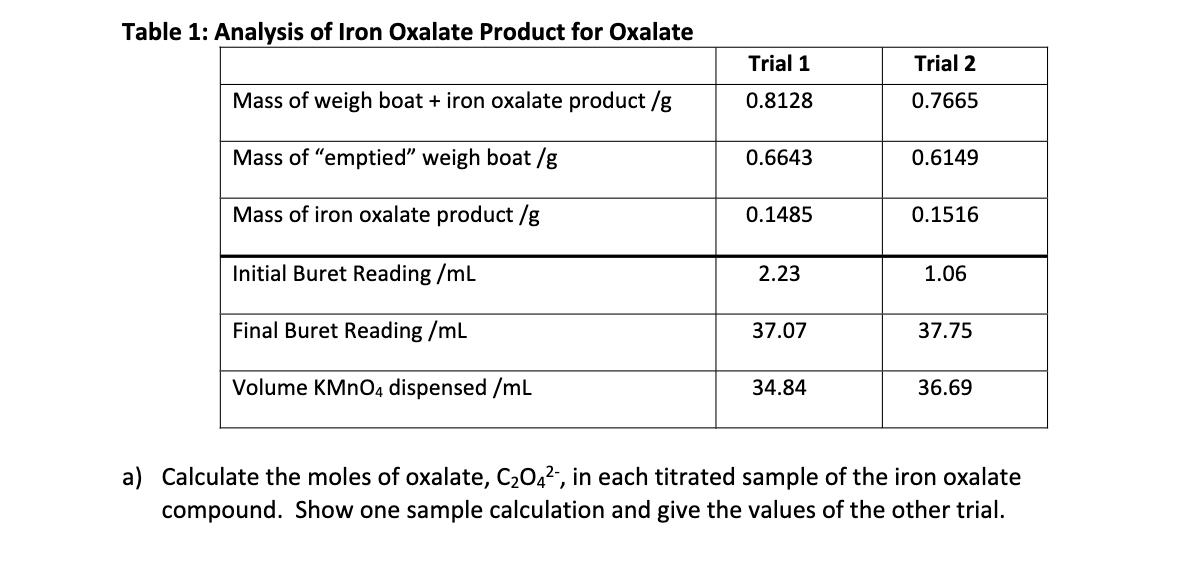# Question Solved1 Answera) Calculate the moles of oxalate, C2O42‐, in each titrated sample of the iron oxalate compound. Show one sample calculation and give the values of the other trial. b) Calculate the average moles of oxalate, C2O42‐, per gram of the iron oxalate compound. UPADATED VALUE AS PER A COMMENT: The molarity of KMnO4 in a solution of 0.0908 g of KMnO4 in 0.500 L of solution is 1.149×10‒3 M. Table 1: Analysis of Iron Oxalate Product for Oxalate Trial 1 Trial 2 Mass of weigh boat + iron oxalate product /g 0.8128 0.7665 Mass of "emptied" weigh boat /g 0.6643 0.6149 Mass of iron oxalate product /g 0.1485 0.1516 Initial Buret Reading /mL 2.23 1.06 Final Buret Reading /mL 37.07 37.75 Volume KMnO4 dispensed /mL 34.84 36.69 a) Calculate the moles of oxalate, C2042, in each titrated sample of the iron oxalate compound. Show one sample calculation and give the values of the other trial.a) Calculate the moles of oxalate, C2O42‐, in each titrated sample of the iron oxalate
compound. Show one sample calculation and give the values of the other trial.

b) Calculate the average moles of oxalate, C2O42‐, per gram of the iron oxalate compound.

UPADATED VALUE AS PER A COMMENT:

The molarity of KMnO4 in a solution of 0.0908 g of KMnO4 in 0.500 L of solution is 1.149×103 M.

Transcribed Image Text: Table 1: Analysis of Iron Oxalate Product for Oxalate Trial 1 Trial 2 Mass of weigh boat + iron oxalate product /g 0.8128 0.7665 Mass of "emptied" weigh boat /g 0.6643 0.6149 Mass of iron oxalate product /g 0.1485 0.1516 Initial Buret Reading /mL 2.23 1.06 Final Buret Reading /mL 37.07 37.75 Volume KMnO4 dispensed /mL 34.84 36.69 a) Calculate the moles of oxalate, C2042, in each titrated sample of the iron oxalate compound. Show one sample calculation and give the values of the other trial.
More
Transcribed Image Text: Table 1: Analysis of Iron Oxalate Product for Oxalate Trial 1 Trial 2 Mass of weigh boat + iron oxalate product /g 0.8128 0.7665 Mass of "emptied" weigh boat /g 0.6643 0.6149 Mass of iron oxalate product /g 0.1485 0.1516 Initial Buret Reading /mL 2.23 1.06 Final Buret Reading /mL 37.07 37.75 Volume KMnO4 dispensed /mL 34.84 36.69 a) Calculate the moles of oxalate, C2042, in each titrated sample of the iron oxalate compound. Show one sample calculation and give the values of the other trial.